# 2nd PUC Basic Maths Question Bank with Answers Karnataka

Karnataka State Board Solutions for 2nd PUC Basic Maths Question Banks with Answers, Solutions, Notes, Guide Pdf are prevailing here in direct pdf links for effective exam preparation. Students who are studying for 2nd PUC Basic Maths textbook solutions can refer to this page for grabbing KSEEB 2nd PUC Basic Maths Solutions pdf chapter wise for free. By reading the textbook solutions. You Can Download 2nd PUC Question Bank with Answers from Karnataka State Board solutions, you will be able to understand all the exercises and score good marks.

Also, there are so many advantages that you can avail from the Karnataka State Board Solutions for 2nd PUC Basic Maths Question Bank with Answers, Solutions pdf. So download 2nd PUC Basic Maths Notes from KTBS Solutions Pdf via quick links & access them easily while preparation. Students can also read 2nd PUC Basic Maths Model Question Papers with Answers hope will definitely help for your board exams.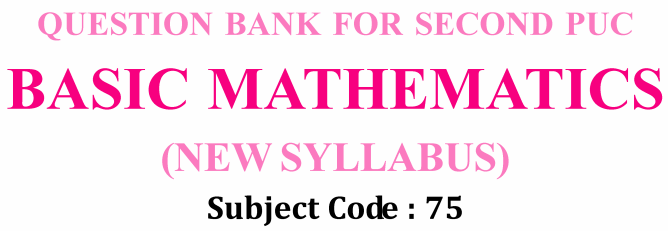## Karnataka 2nd PUC Maths Question Bank with Answers

Provided Karnataka State Board solutions for 2nd PUC Basic Maths textbook solutions are prepared by subject experts after ample research. So students are suggested to download the 2nd PUC Basic Maths Question Bank with Answers and Textbook Solutions from the available PDF links and kickstart your preparations for final exams. You can download them freely & practice regularly to score high marks in the examinations. Access the below Chapter-wise links and learn complete by using 2nd PUC Basic Maths Question Bank with Answers from Karnataka State Board solutions pdf.

2nd PUC Basic Maths Question Bank Chapter 1 Matrices and Determinants

2nd PUC Basic Maths Question Bank Chapter 2 Permutations and Combinations

2nd PUC Basic Maths Question Bank Chapter 3 Probability

2nd PUC Basic Maths Question Bank Chapter 4 Binomial Theorem

2nd PUC Basic Maths Question Bank Chapter 5 Partial Fractions

2nd PUC Basic Maths Question Bank Chapter 6 Mathematical Logic

2nd PUC Basic Maths Question Bank Chapter 7 Ratios and Proportions

2nd PUC Basic Maths Question Bank Chapter 8 Bill Discounting

2nd PUC Basic Maths Question Bank Chapter 9 Stocks and Shares

2nd PUC Basic Maths Question Bank Chapter 10 Learning Curve

2nd PUC Basic Maths Question Bank Chapter 11 Linear Programming Problems

2nd PUC Basic Maths Question Bank Chapter 12 Sales Tax and Value Added Tax

2nd PUC Basic Maths Question Bank Chapter 13 Heights and Distances

2nd PUC Basic Maths Question Bank Chapter 14 Compound Angles, Multiple Angles, Sub Multiples Angles and Transformation Formulae

2nd PUC Basic Maths Question Bank Chapter 15 Circles

2nd PUC Basic Maths Question Bank Chapter 16 Parabola

2nd PUC Basic Maths Question Bank Chapter 17 Limits and Continuity of a Function

2nd PUC Basic Maths Question Bank Chapter 18 Differential Calculus

2nd PUC Basic Maths Question Bank Chapter 19 Application of Derivatives

2nd PUC Basic Maths Question Bank

2nd PUC Basic Maths Question Bank Chapter 21 Definite Integrals and Its Application to Areas

Karnataka 2nd PUC Maths Blue Print of Model Question Paper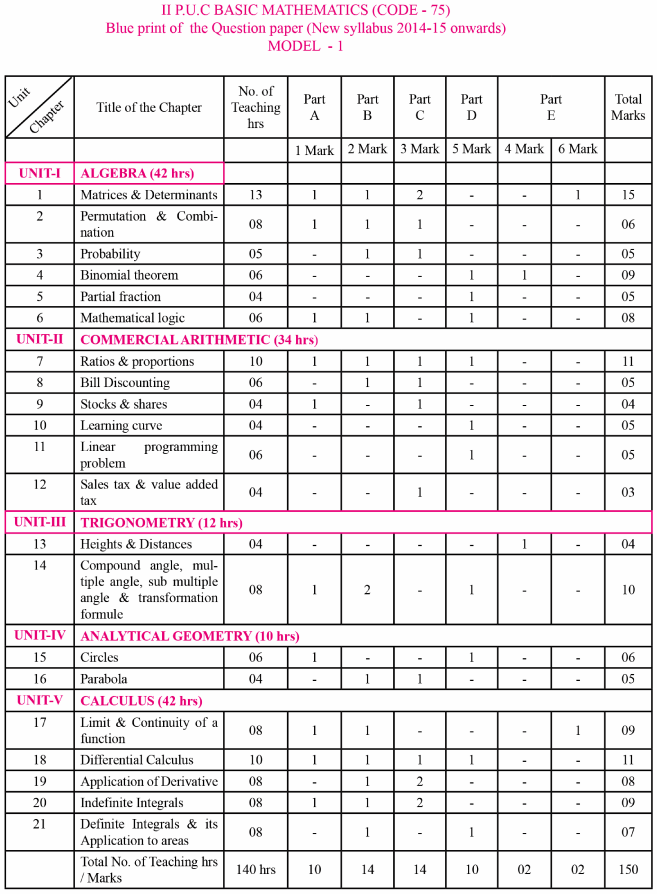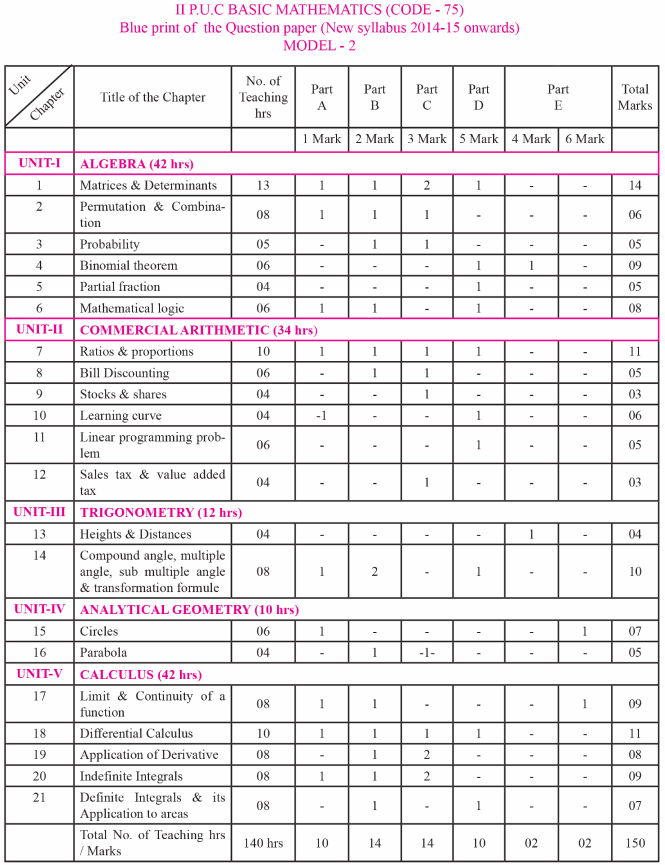2nd PUC Basic Mathematics Weightage Given To The Curriculum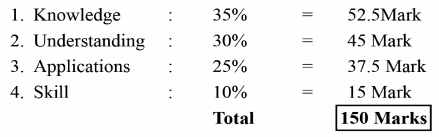2nd PUC Basic Mathematics Unit Wise Weightage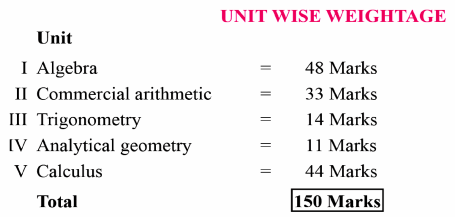Instruction To Question Paper Setters

Note: In The Chapter (4) Binomial Theorem The Proof Of The Binomial Theorem For Positive Integral Power Is Excluded
PART – E

6 Marks Questions Must Be Selected From The Following Topics Only:

1. Application of matrix: (3 x 3) order about statement problem, formation of linear equation and solve them by matrix method
2. Circle: Problems on concylic (circle passes through 4 points)
3. Limits: standard theorem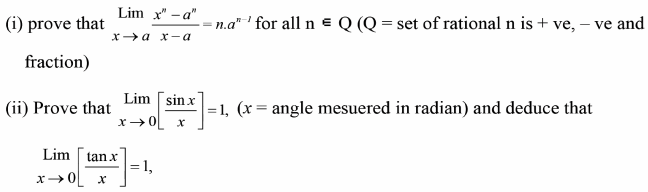4 Marks Question Must Be Selected From The Following Topic Only.

1. Binomial theorem: Application problems like Evaluate : (0. 98)5, (1.01)5, (102)4, (0.97)4… etc upto 4 decimal.
2. Heights and distances : Application problems
3. Linear programming problem (L.P.P): Statement problems on L.P.P for mationtion of linear equations.
4. Cost and revenue function : Problems on total cost, total revenue, Marginal cost, Marginal revenue, Profit Maximization etc.

Model Question Paper Pattern
Instructions:

1. The question paper has 5 parts A, B, C, D & E. Answer all the parts.
2. Part A carries 10 marks, Part B carries 20 marks, part C carries 30 marks, Part D carries 30 marks and part E carries 10 marks.
3. Write the question numbers properly as indicated in the question paper.

We wish the data shed above will benefit you at the time of your preparation & gain good scores. So, download Karnataka State Board 2nd PUC Basic Maths Question Bank with Answers from here and become a topper in the final examinations. Moreover, keep in touch with our site & get all updated chapter wise KSEEB State Board Solutions for all subjects.